American Journal of Theoretical and Applied Statistics
Volume 5, Issue 2-1, March 2016, Pages: 7-11

Efficient Approach to Pattern Recognition Based on Minimization of Misclassification Probability

Nicholas A. Nechval1, *, Konstantin N. Nechval2

1Department of Mathematics, Baltic International Academy, Riga, Latvia

2Department of Applied Mathematics, Transport and Telecommunication Institute, Riga, Latvia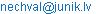(N. A. Nechval)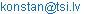(K. N. Nechval)

Nicholas A. Nechval, Konstantin N. Nechval. Efficient Approach to Pattern Recognition Based on Minimization of Misclassification Probability. American Journal of Theoretical and Applied Statistics. Special Issue: Novel Ideas for Efficient Optimization of Statistical Decisions and Predictive Inferences under Parametric Uncertainty of Underlying Models with Applications. Vol. 5, No. 2-1, 2016, pp. 7-11. doi: 10.11648/j.ajtas.s.2016050201.12

Abstract: In this paper, an efficient approach to pattern recognition (classification) is suggested. It is based on minimization of misclassification probability and uses transition from high dimensional problem (dimension p≥2) to one dimensional problem (dimension p=1) in the case of the two classes as well as in the case of several classes with separation of classes as much as possible. The probability of misclassification, which is known as the error rate, is also used to judge the ability of various pattern recognition (classification) procedures to predict group membership. The approach does not require the arbitrary selection of priors as in the Bayesian classifier and represents the novel pattern recognition (classification) procedure that allows one to take into account the cases, which are not adequate for Fisher’s classification rule (i.e., the distributions of the classes are not multivariate normal or covariance matrices of those are different or there are strong multi-nonlinearities). Moreover, it also allows one to classify a set of multivariate observations, where each of the observations belongs to the same unknown class. For the cases, which are adequate for Fisher’s classification rule, the proposed approach gives the results similar to that of Fisher’s classification rule. For illustration, practical examples are given.

Keywords: Pattern, Recognition, Classification, Misclassification, Probability, Minimization

1. Introduction

Pattern recognition aim is to classify data (patterns) based on statistical information extracted from the patterns [1,2]. It provides the solution to various problems from speech recognition, face recognition to classification of handwritten characters and medical diagnosis. The various application areas of pattern recognition are like bioinformatics, document classification, image analysis, data mining, industrial automation, biometric recognition, remote sensing, handwritten text analysis, medical diagnosis, speech recognition, statistics, diagnostics, computer science, biology and many more. Pattern recognition aim is to classify data (patterns) based on either a priori knowledge or on statistical information extracted from the patterns. Fisher’s linear discriminant rule (FLDR) is the most widely used classification rule [3-9 and references therein]. Some of the reasons for this are its simplicity and unnecessity of strict assumptions. In its original form, proposed by Fisher, the method assumes equality of population covariance matrices, but does not explicitly require multivariate normality. However, optimal classification performance of Fisher's discriminant function can only be expected when multivariate normality is present as well, since only good discrimination can ensure good allocation. In practice, we often are in need of analyzing input data samples, which are not adequate for Fisher’s classification rule, such that the distributions of the groups (classes, populations) are not multivariate normal or covariance matrices of those are different or there are strong multi-nonlinearities.

In this paper, an efficient approach to pattern recognition (classification) is proposed. It is based on minimization of misclassification probability. The approach does not require the arbitrary selection of priors as in the Bayesian classifier and represents the novel procedure that allows one to analyze input data samples, which are not adequate for Fisher’s pattern classification rule (i.e., the distributions of the classes are not multivariate normal or covariance matrices of those are different or there are strong multi-nonlinearities). For the cases, which are adequate for Fisher’s classification rule, the proposed approach gives the results similar to that of Fisher’s rule. Moreover, it also allows one to classify the set of multivariate observations, where each of the observations belongs to the same class. This approach uses transition from high dimensional problem (dimension p³2) to one dimensional problem (dimension p=1) in the case of the two classes as well as in the case of several classes with separation of classes as much as possible. The probability of misclassification, which is known as the error rate, is also used to judge the ability of various pattern recognition (classification) procedures to predict group membership.

2. Approach to Pattern Recognition

2.1. Approach to Pattern Recognition (Classification) in the Case of Two Classes

Let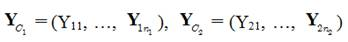(1)

be samples of observed vectors of attributes of objects from two different classes C1 and C2, respectively. In this case, the proposed approach to pattern recognition (classification) is as follows.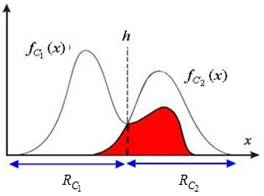Figure 1. Misclassification probability.

Step 1 (Transition from high dimensional problem (dimension p³2) to one dimensional problem (dimension p=1)). At this step, transition from high dimensional problem to one dimensional problem is carried out by using suitable transformations of the multivariate (p³2) observations Y = [Y1,…, Yp]′ to univariate observations X with separation of classes as much as possible to obtain the input object allocation, which should be "optimal" in the sense of minimizing, on average, the number of incorrect assignments. Then the separation threshold h, which minimizes the probability of misclassification of the new input observation Ynew,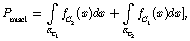(2)

is determined (see Fig. 1), where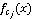represents the probability density function (pdf) of a transformed observation X= X (Ynew) of Ynew from class Cj, jÎ{1, 2}.

Step 2 (Pattern recognition (classification) via separation threshold h). At this step, pattern recognition (classification) of the new observation Ynew is carried out as follows: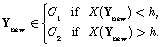(3)

Remark 1. The recognition (classification) rule (3) can be rewritten as follows:

Assign Ynew to the class Cj for which, jÎ{1, 2}, is largest.

2.2. Approach to Pattern Recognition (Classification) in the Case of Several Classes

Let(4)

be samples of observed vectors of objects from several different classes C1, C2, …, Cm, respectively. In this case, the proposed approach to pattern recognition (classification) is as follows.

Step 1 (Transition from high dimensional problem (dimension p³2) to one dimensional problem (dimension p=1)). At this step, at first, transition from p-dimensional problem to g-dimensional problem is carried out by using a suitable transformation of the multivariate observations Y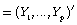to the multivariate observations Z=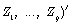, where g must be no bigger  than(5)

If g³2, transition from g-dimensional problem to one dimensional problem is carried out by using a suitable transformation of the multivariate observations Z= (to univariate observations X with separation of classes as much as possible to obtain the input object allocation, which should be "optimal" in the sense of minimizing, on average, the number of incorrect assignments. Then the separation threshold hkl, which minimizes the probability of misclassification of the new input observation Ynew for classes Ck and Cl,

k, lÎ{1, …, m}, k¹l,             (6)

is determined (pairwise), whererepresents the pdf of a transformed observation X(Ynew) from class Ck.

Step 2 (Pattern recognition (classification) via separation thresholds hkl; k, lÎ{1, …, m}, k¹l). At this step, pattern recognition (classification) of the new observation Ynew is carried out as follows: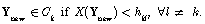(7)

Remark 2. The recognition (classification) rule (7) can be rewritten as follows: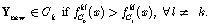(8)

3. Practical Examples

3.1. Example 1

Suppose we wish to classify some product (input vector Y = [Y1, Y2]¢ ) to one of two classes of quality (C1 and C2) of this product. The data samples of observed vectors Y of attributes of product quality from two different classes C1 and C2, respectively, are given in Table 1.

Table 1. Product quality attributes data.

 Class C1 of product quality attributes Vector Y′1(i) of quality attributes i y11(i) y12(i) i y11(i) y12(i) 1. 6 6.8 9. 7.5 5.3 2. 5.8 6.8 10. 6.8 5 3. 6.3 7 11. 5 4.4 4. 7 6.3 12. 5.7 4.6 5. 6.4 5.9 13. 7.1 4.1 6. 7.7 5.9 14. 7.8 4.3 7. 5 5.7 15. 6.1 3.9 8. 6.1 5.2 Class C2 of product quality attributes Vector Y′2(i) of quality attributes i y21(i) y22(i) i y21(i) y22(i) 1. 4.2 9.4 10. 10.3 5 2. 6.9 9 11. 11.7 4.4 3. 8.7 9 12. 3.5 3.7 4. 4.9 8.4 13. 9.2 3.2 5. 3.4 7.6 14. 7.4 2.8 6. 11.2 7.5 15. 4.2 2.2 7. 9.2 6.3 16. 9 2.3 8. 3.1 6 17. 11 2 9. 1.8 4.9 18. 5.9 1.8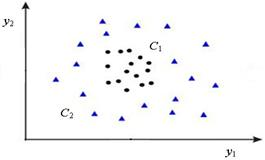Figure 2. Pictorial representation of the data of Table 1, which are not adequate for Fisher’s classification rule.

A pictorial representation of the above data, which are not adequate for Fisher’s classification rule, is given on Fig. 2. If the points are projected in any direction onto a straight line, there will be almost total overlap. A linear discriminant procedure will not successfully separate the two classes.

Step 1. For transition from high dimensional problem (p=2) to one dimensional problem (p=1), the following transformations are used: Y=[Y1, Y2]¢ Þ Z=[Z1, Z2, Z3]¢ Þ X, where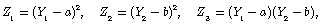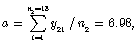(9)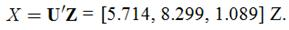(10)

Using the Anderson-Darling goodness-of-fit test for Normality (significance level a=0.05), it was found that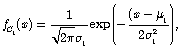(11)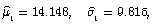(12)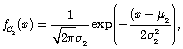(13)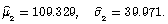(14)

It follows from (2) that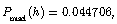(15)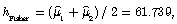(16)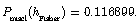(17)

Indexes. Thus, the index of relative efficiency of the Fisher approach as compared with the proposed approach is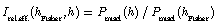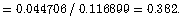(18)

The index of reduction percentage in the probability of misclassification for the proposed approach as compared with the Fisher approach is given by(19)

3.2. Example 2

This example is adapted from a study  concerned with the detection of hemophilia A carriers. To construct a procedure for detecting potential hemophilia A carriers, blood samples were assayed for two groups of women and measurements on the two variables: Y1=log10(AHF activity) and Y2=log10(AHF antigen) recorded. ("AHF" denotes antihemophilic factor.) The first group of n1 =23 women was selected from known hemophilia A carriers. This group was called the obligatory carriers. The second group of n2=29 women were selected from a population of women who did not carry the hemophilia gene. This group was called the normal group. The pairs of observations (y1, y2) for the two groups are given in Table 2 and plotted in Fig. 3. Also shown are estimated contours con­taining 50% and 95% of the probability for bivariate normal distributions cen­tered at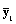and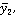respectively.

Table 2. Hemophilia data.

 Group C1 of obligatory carriers Vector Y′1(i) = [log10(AHF activity, log10(AHF antigen] i y11(i) y12(i) i y11(i) y12(i) 1. -0.45 0.015 13. -0.25 -0.04 2. -0.43 -0.095 14. -0.22 -0.015 3. -0.42 -0.12 15. -0.22 0.024 4. -0.41 -0.25 16. -0.21 -0.04 5. -0.38 -0.28 17. -0.175 -0.09 6. -0.35 -0.015 18. -0.2 0.25 7. -0.34 0.1 19. -0.19 0.175 8. -0.33 -0.13 20. -0.075 0.17 9. -0.24 0.28 21. -0.015 0.15 10. -0.24 0.15 22. -0.07 0.0135 11. -0.26 0.08 23. -0.025 0.08 12. -0.26 -0.075 Group C2 of noncarriers Vector Y′2(i) = [log10(AHF activity, log10(AHF antigen] i y21(i) y22(i) i y21(i) y22(i) 1. -0.23 -0.3 16. 0.03 0.09 2. -0.18 -0.3 17. 0.05 0 3. -0.13 -0.3 18. 0.04 -0.03 4. -0.16 -0.24 19. 0.1 0 5. -0.025 -0.2 20. 0.075 0.02 6. -0.12 -0.14 21. 0.055 0.05 7. -0.075 -0.14 22. 0.06 0.1 8. -0.02 -0.15 23. 0.09 0.09 9. -0.07 -0.06 24. 0.1 0.05 10. -0.06 -0.055 25. 0.11 0.035 11. -0.025 -0.09 26. 0.1 0.125 12. -0.06 -0.04 27. 0.12 0.125 13. 0 -0.08 28. 0.14 0.07 14. 0.05 -0.08 29. 0.21 0.11 15. 0.07 -0.1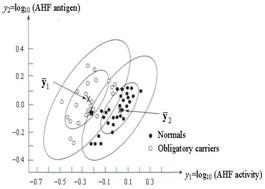Figure 3. Scatter plots of [log10 (AHF activity), log10(AHF antigen)] for the normal group and obligatory hemophilia A carriers.

Step 1. For transition from high dimensional problem (p=2) to one dimensional problem (p=1), the following transformation is used: Y=[Y1 Y2]¢ Þ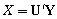, where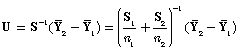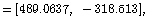(20)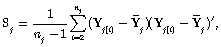(21)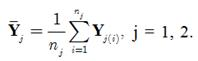(22)

Using the Anderson-Darling goodness-of-fit test for Normality (significance level a=0.05), it was found that(23)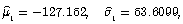(24)(25)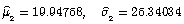(26)

Theorem 1. If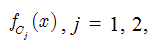(27)

are the probability density functions of the normal distribution with the parameters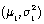and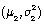, respectively, where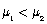, then the necessary and sufficient conditions for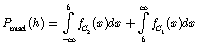(28)

to have a unique minimum are:

(i) the necessary condition for h to be a minimum point of (5) is given by(29)

(ii) the sufficient condition for h to be a minimum point of (28) is given by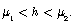(30)

Proof. The proof being straightforward is omitted here.

Corollary 1.1. If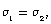then the separation threshold h is determined as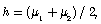(31)

i.e., in this case we deal with Fisher’s separation threshold.

It follows from (28) that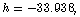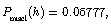(32)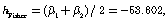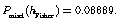(33)

Indexes. Thus, the index of relative efficiency of the Fisher approach as compared with the proposed approach is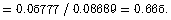(34)

The index of reduction percentage in the probability of misclassification for the proposed approach as compared with the Fisher approach is given by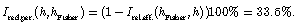(35)

Step 2 (Pattern recognition (classification) via separation threshold h). At this step, pattern recognition (classification) of a new observation Ynew is carried out as follows: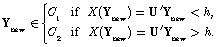(36)

For instance, measurements of AHF activity and AHF antigen on a woman who may be a hemophilia A carrier give y1 = -0.210 and y2 = -0.044. Should this woman be classified as C1 (obligatory carrier) or C2 (normal)?

Using Fisher’s classification rule, we obtain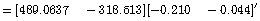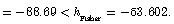(37)

Using the proposed approach based on minimization of misclassification probability, we obtain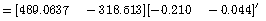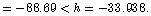(38)

Applying either (37) or (38), we classify the woman as C1, an obligatory carrier. Thus, Fisher’s approach and the proposed one give the same result in the above case.

It will be noted that if Ynew=Y1(22)=[ -0.07 0.0135]¢, then Xnew= -38.5344 > hFisher= -53.602. Thus, in this case, Fisher’s classification rule gives incorrect classification.

4. Conclusion

The approach proposed in this paper represents the innovative pattern recognition (classification) procedure based on minimization of misclassification probability. It allows one to take into account the cases, which are not adequate for Fisher’s classification rule. Moreover, the procedure also allows one to classify the set of multivariate observations, where each of the observations belongs to the same unknown class. This approach has been motivated by a misclassification problem that appears in various application areas of pattern recognition.

References

1. R. Fisher, "The use of multiple measurements in taxonomic problems," Ann. Eugenics, vol. 7, pp. 178-188, 1936.
2. K. V. Mardia, J. T. Kent, and J. M. Bibby, Multivariate Analysis. Academic Press, 1979.
3. N. A. Nechval, K. N. Nechval, and M. Purgailis, "Statistical pattern recognition principles," in International Encyclopedia of Statistical Science, Part 19, Miodrag Lovric, Ed. Berlin, Heidelberg: Springer-Verlag, 2011, pp. 1453-1457.
4. N. A. Nechval, K. N. Nechval, M. Purgailis, V. F. Strelchonok, G. Berzins, and M. Moldovan, "New approach to pattern recognition via comparison of maximum separations," Computer Modelling and New Technologies, vol. 15, pp. 30-40, 2011.
5. N. A. Nechval, K. N. Nechval, V. Danovich, G. Berzins, "Distance-based approaches to pattern recognition via embedding," in Lecture Notes in Engineering and Computer Science: Proceedings of The World Congress on Engineering 2014, 2-4 July, 2014, London, U.K., pp. 759-764.
6. R.O. Duda, P. E. Hart,and D. G. Stork, Pattern classification. New York: Wiley.(Second Edition.),2001.
7. S. T. John and C. Nello, Kernel Methods for Pattern Analysis. Cambridge: Cambridge University Press, 2004.
8. A. C. Rencher, Methods of Multivariate Analysis. John Wiley & Sons. (Second Edition.), 2002.
9. T. Sergios and K. Konstantinos, Pattern Recognition. Singapore: Elsevier Ltd. (Third Edition.), 2006.
10. B. N. Bouma, et al.,Evaluation of the detection rate of hemophilia carriers. Statistical Methods for Clinical Decision Making, vol. 7, pp. 339-350, 1975.

 Contents 1. 2. 2.1. 2.2. 3. 3.1. 3.2. 4.
Article ToolsAbstractPDF(438K)